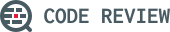## How Rich Are the 1% (Active Users)

0

(click on this box to dismiss)

Occupy Stack Overflow!Q&A for peer programmer code reviews

``````-- How Rich Are the 1% (Active Users)
-- Occupy Stack Overflow!

;WITH percentile AS (
SELECT NTILE(100) OVER (ORDER BY Reputation DESC) as p,
Reputation,
DisplayName
FROM Users
WHERE Reputation >= 200
),
total AS (
SELECT SUM(Reputation) as total
FROM percentile
)
SELECT
'The ' + CASE p WHEN 1 THEN '1' ELSE '99' END + '%' AS Who,
SUM(Reputation) AS Rep,
COUNT(*) AS Users,
CAST(SUM(Reputation) AS DECIMAL(19,2)) / total.total * 100 AS PctShareOfWealth,
ROUND(CAST(SUM(Reputation) AS DECIMAL(19,2)) / COUNT(*),2) AS AvgUserRep
FROM percentile, total
GROUP BY CASE p WHEN 1 THEN '1' ELSE '99' END, total.total``````

### Enter Parameters

Options:
-Hold tight while we fetch your results
:records returned in :time ms:cached